Next: Discussion Up: tr05mj1 Previous: Performance Criteria

# Results

Some sample plots of the similarity functions,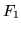and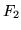are shown in figure 4. These show the functions over a relatively large range of translations, and demonstrate two important features: that the secondary (incorrect) peak is downweighted in, reducing the chances large mis-matches; and in the close-up results, the improved continuity of the similarity functions (which is smoother and has less discontinuities for).

Figure 4: Example similarity functions for(left),(middle) and(right). The top row shows the functions over a large range of translations, the second and third rows show a close-up of the functions, with the actual calculated values represented by circles. The true translation value is -10.2mm for all of these plots.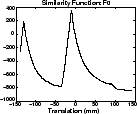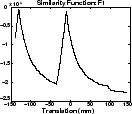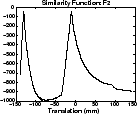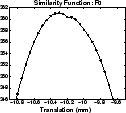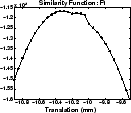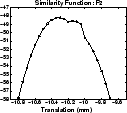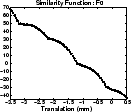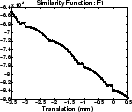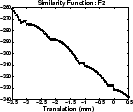Table 1 shows the values of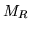and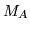for 3 different SNR values and 2000 trials in each case. The low values offorare a result of the downweighting of the secondary maxima, significantly reducing bad mismatches. Also,was the most accurate (having the smallest value of) which is likely to be due to the decrease in discontinuities in.

Table 1: Results of robustness and accuracy measures for a simple object (see figure 3b) and a range of SNR values, for each of the three cost functions.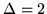mm.
 SNR = 5 SNR = 15 SNR = 50MR = 0.194 MA = 0.71 MR = 0.065 MA = 0.26 MR = 0.000 MA = 0.20MR = 1.000 - MR = 0.909 MA = 0.35 MR = 0.807 MA = 0.97MR = 0.516 MA = 0.86 MR = 0.494 MA = 0.48 MR = 0.129 MA = 0.54Next: Discussion Up: tr05mj1 Previous: Performance Criteria